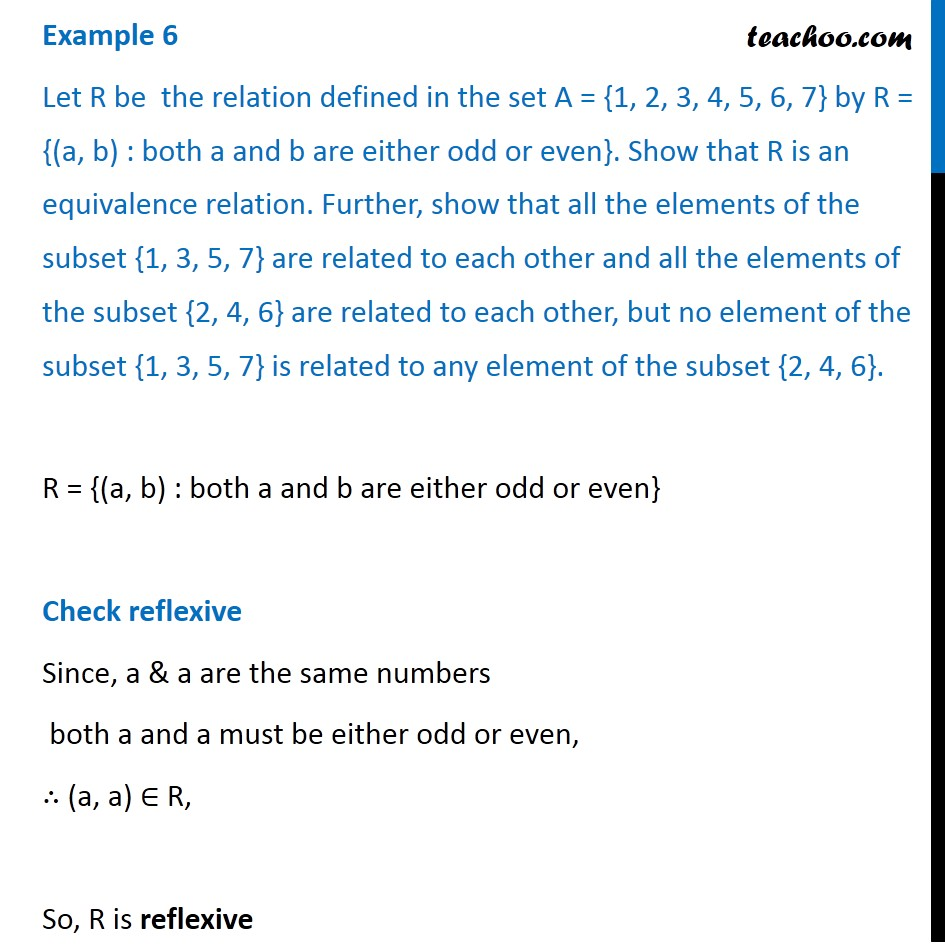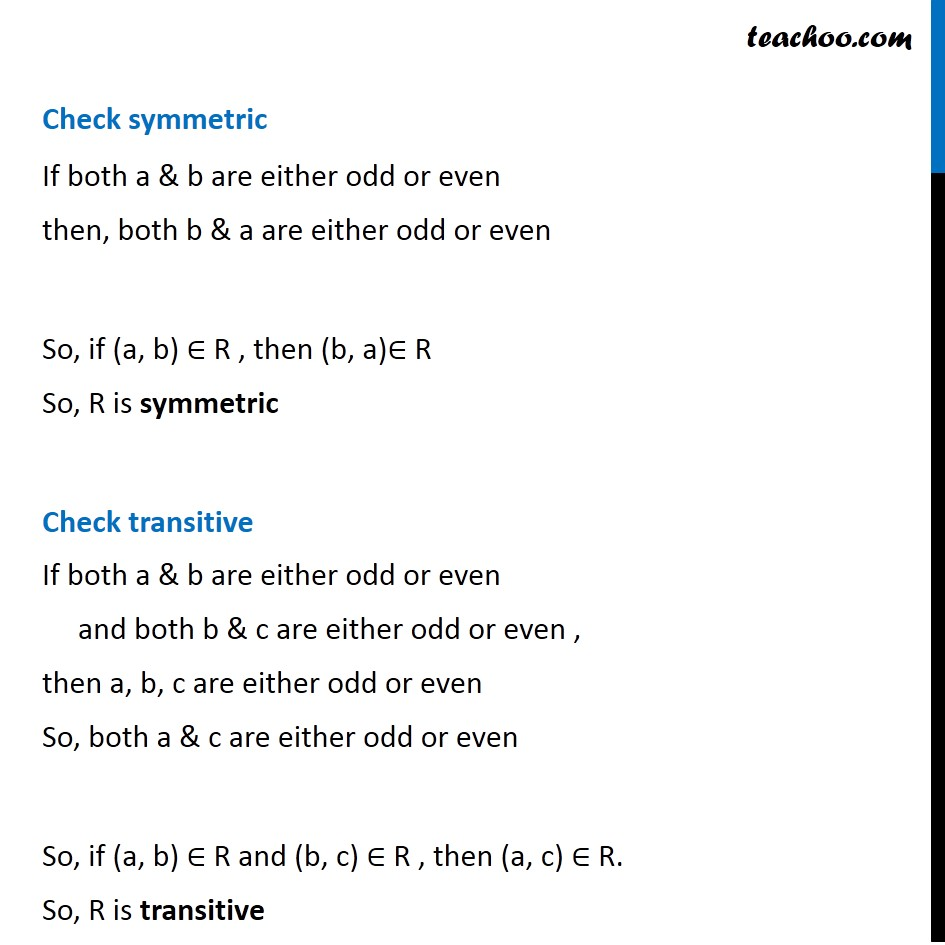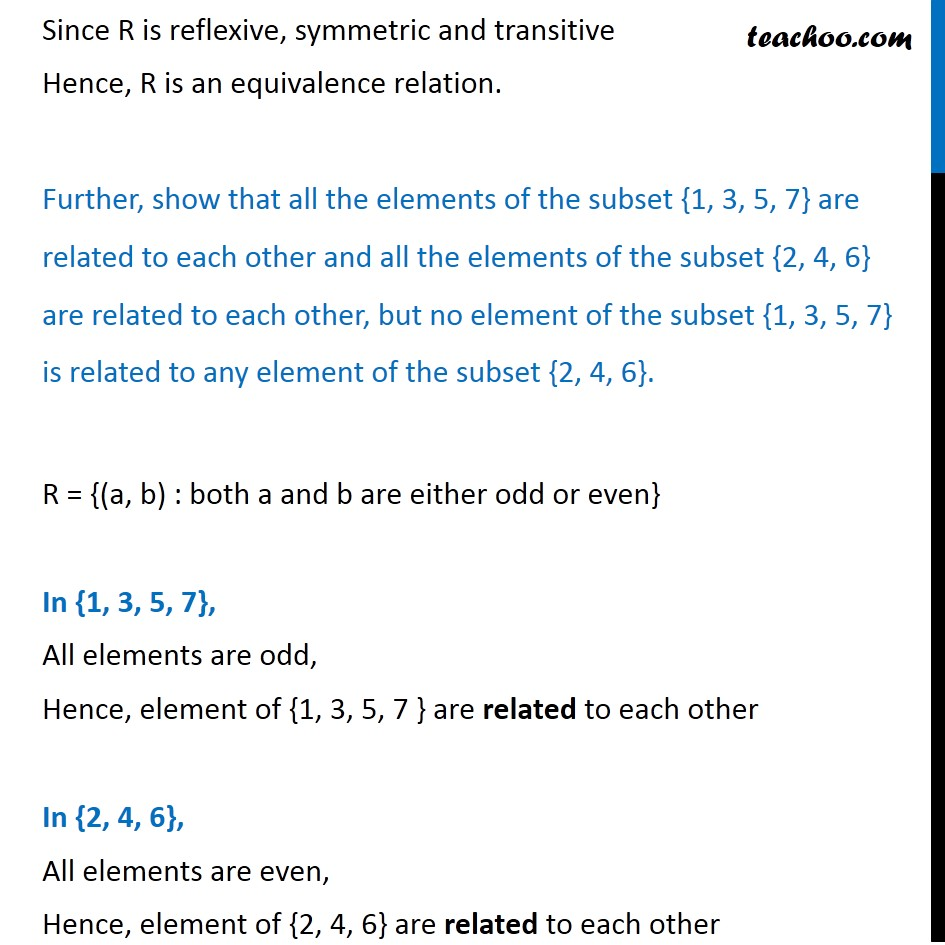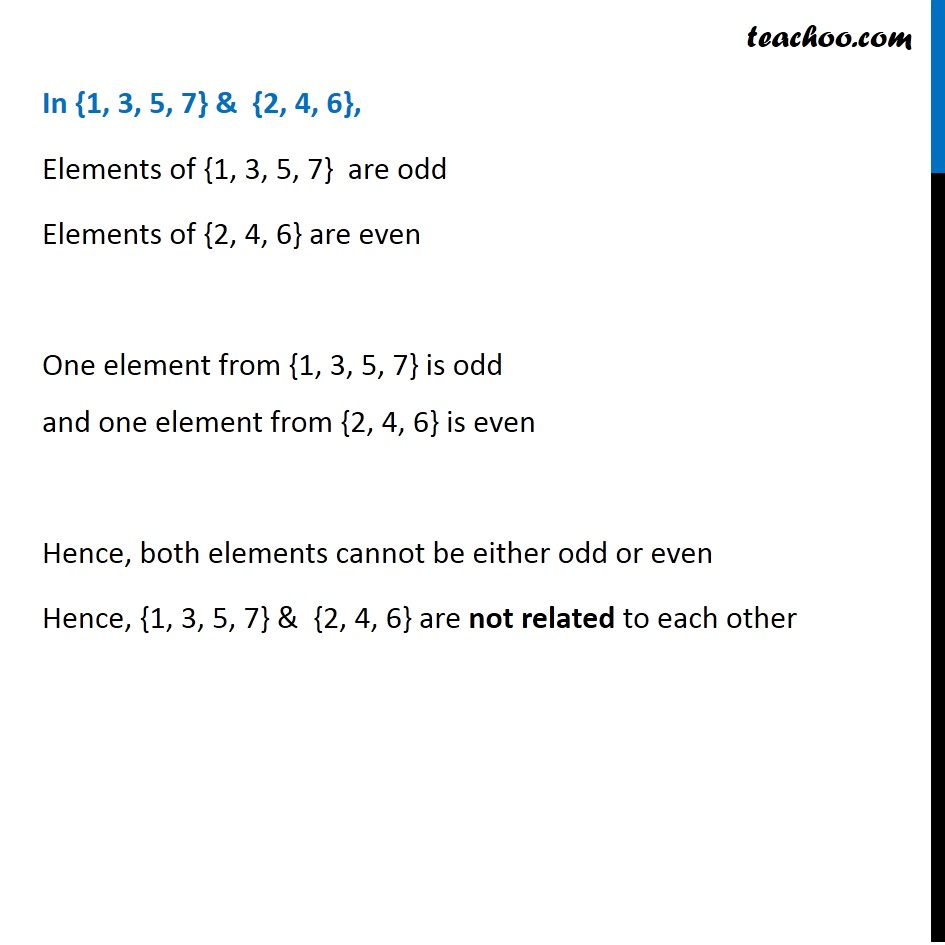To prove relation reflexive, transitive, symmetric and equivalent

Chapter 1 Class 12 Relation and Functions
Concept wiseIntroducing your new favourite teacher - Teachoo Black, at only ₹83 per month

### Transcript

Example 6 Let R be the relation defined in the set A = {1, 2, 3, 4, 5, 6, 7} by R = {(a, b) : both a and b are either odd or even}. Show that R is an equivalence relation. Further, show that all the elements of the subset {1, 3, 5, 7} are related to each other and all the elements of the subset {2, 4, 6} are related to each other, but no element of the subset {1, 3, 5, 7} is related to any element of the subset {2, 4, 6}. R = {(a, b) : both a and b are either odd or even} Check reflexive Since, a & a are the same numbers both a and a must be either odd or even, ∴ (a, a) ∈ R, So, R is reflexive Check symmetric If both a & b are either odd or even then, both b & a are either odd or even So, if (a, b) ∈ R , then (b, a)∈ R So, R is symmetric Check transitive If both a & b are either odd or even and both b & c are either odd or even , then a, b, c are either odd or even So, both a & c are either odd or even So, if (a, b) ∈ R and (b, c) ∈ R , then (a, c) ∈ R. So, R is transitive Since R is reflexive, symmetric and transitive Hence, R is an equivalence relation. Further, show that all the elements of the subset {1, 3, 5, 7} are related to each other and all the elements of the subset {2, 4, 6} are related to each other, but no element of the subset {1, 3, 5, 7} is related to any element of the subset {2, 4, 6}. R = {(a, b) : both a and b are either odd or even} In {1, 3, 5, 7}, All elements are odd, Hence, element of {1, 3, 5, 7 } are related to each other In {2, 4, 6}, All elements are even, Hence, element of {2, 4, 6} are related to each other In {1, 3, 5, 7} & {2, 4, 6}, Elements of {1, 3, 5, 7} are odd Elements of {2, 4, 6} are even One element from {1, 3, 5, 7} is odd and one element from {2, 4, 6} is even Hence, both elements cannot be either odd or even Hence, {1, 3, 5, 7} & {2, 4, 6} are not related to each other